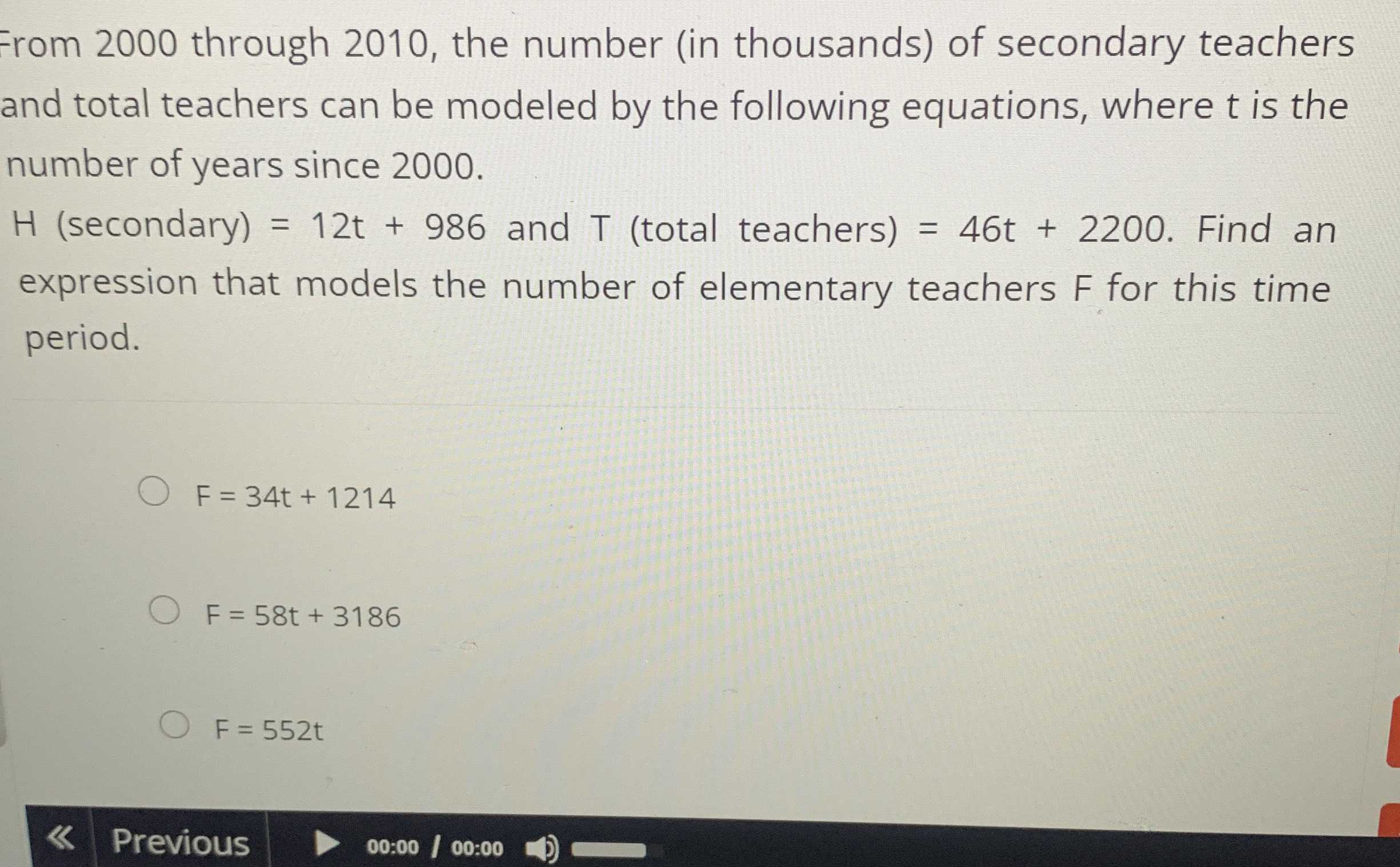### Still have math questions?

Algebra
QuestionGrom $$2000$$ through $$2010$$ , the number (in thousands) of secondary teachers and total teachers can be modeled by the following equations, where $$t$$ is the number of years since $$2000$$ .

$$H$$ (secondary) $$= 12 t + 986$$ and $$T$$ (total teachers) $$= 46 t + 2200$$ . Find an expression that models the number of elementary teachers $$F$$ for this time period.

$$F = 34 t + 1214$$

$$F = 58 t + 3186$$